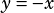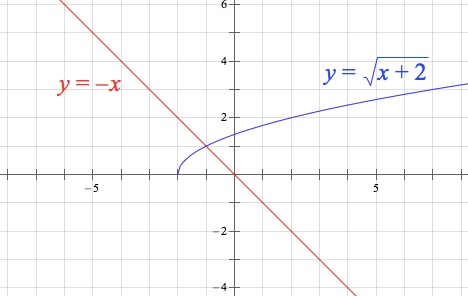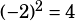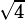What is the set of all solutions to the equation square root of (x+2) = -x?

A) (-1,2) B) (-1), C) (2), D) There are no solutions.

Answer is (-1), but why won’t (-1,2) work? Square root of (2+2) = √4 = +/- 2, right?

Unfortunatey, 2 is what’s called an extraneous solution, which we’re in danger of creating any time we square both sides of an equation in order to solve. I know this isn’t a calculator-allowed question, but a graph is a good illustrator of this concept. If you graph bothand, you’ll see all the solutions to the equation above:First, backsolving is a great way to go. Often, as in this case, there are only two actual numbers to try (–1 and 2, here) and the answer choices are just combinations of those.Second, remember that the square root radical symbol (√) denotes the positive square root. I don’t know who made this rule, but I believe it’s because the square root needs to be a function, and a function can only have one output per input (otherwise it would fail the vertical line test). So while it’s true that, it’s not the case that. When you see, you should only be thinking positive 2.

Finally, the algebra in case anyone reading this is allergic to score improvement via backsolving. 🙂From there it certainly looks like the solutions are –1 and 2, but because we squared the equation in the path to our solution, we must check for extraneous solutions. We do this by dropping the possible solutions back into the original equation (before we squared it). So I’m just going to copy and paste from above because when you backsolve you START with this step:Again we see that –1 works, but 2 does not.